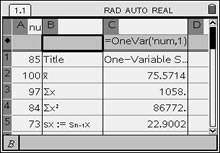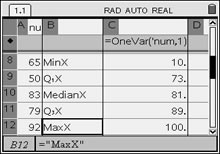Five Number Summary

The "five number summary", or five statistical summary", consists of
(1) the minimum, (2) the maximum, (3) the median, (4) the first quartile
and (5) the third quartile.

Find the "five number summary" for the data set
{85, 100, 97, 84, 73, 89, 73, 65, 50, 83, 79, 92, 78, 10}.

Method 1.   A box and whisker plot will clearly show a five number summary.  See box-and-whiskers for information on creating these graphs.

Pressing the TRACE key will display the values.  The whiskers extend from the minimum data point in the set to the first quartile, and from the third quartile to the maximum point.   The box itself is defined by Q1, the median and Q3.  The spider will jump from the minimum value to Q1, to median, to Q3 and to the maximum value.Method 2:  A complete five number summary is displayed on the lower portion of the One-Variable Statistics screen.

Enter the data on a List & Spreadsheet Page.
This list is named "num".

Press, #4 Statistics, #1 Stat Calculations, and choose #1 One-Variable Statistics.

The choice "Open Variable Statistics" yields the following results back to the spreadsheet columns. One column will "label" these values, while the following column contains the actual values. The five number summary information is contained within these results.= mean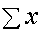= the sum of the data= the sum of the squares of the data sx = the sample standard deviation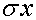= the population standard deviation n = the sample size (# of pieces of data) MinX = the smallest data entry (minimum)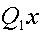= data at the first quartile MedianX = data at the median (second quartile)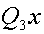= data at the third quartile MaxX = the largest data entry (maximum) SSx = the sum of squared deviations of x from the    mean of x.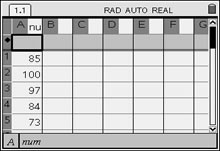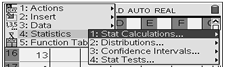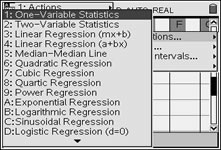When prompted for the number of lists, enter 1. When promted for X1 list enter the name of the list and frequency 1. Widen the columns. Arrow down to see the five number summary.